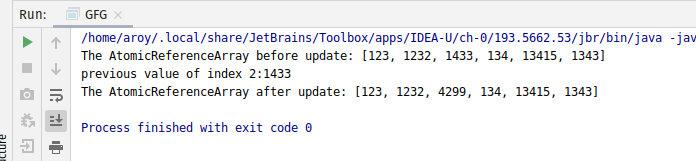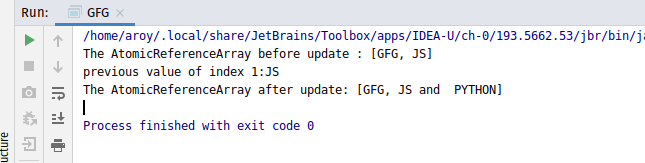# AtomicReferenceArray getAndAccumulate() method in Java with Examples

• Last Updated : 03 Jan, 2020

The getAndAccumulate() method of a AtomicReferenceArray class is used to atomically updates the element at index i of AtomicReferenceArray with the results of applying the given accumulatorFunction to the current and given values and returns the previous value. The accumulatorFunction should be side-effect-free, since it may be re-applied when attempted updates fail due to contention among threads. The function is applied with the current value at index i as its first argument, and the given update as the second argument.

Syntax:

```public final E getAndAccumulate(int i, E x,
BinaryOperator<E> accumulatorFunction)

```

Parameters: This method accepts:

• i which is an index of AtomicReferenceArray to perform the operation accepts,
• x which is the updated value and
• accumulatorFunction which is a side-effect-free function of two arguments.

Return value: This method returns the previous value.

Below programs illustrate the getAndAccumulate() method:
Program 1:

 `// Java program to demonstrate``// getAndAccumulate() method`` ` `import` `java.util.concurrent.atomic.*;``import` `java.util.function.BinaryOperator;`` ` `public` `class` `GFG {``    ``public` `static` `void` `main(String args[])``    ``{``        ``// an array``        ``Integer a[]``            ``= { ``123``, ``1232``, ``1433``, ``134``, ``13415``, ``1343` `};`` ` `        ``// AtomicReferenceArray with array``        ``AtomicReferenceArray array``            ``= ``new` `AtomicReferenceArray<>(a);`` ` `        ``// Print AtomicReferenceArray``        ``System.out.println(``            ``"The AtomicReferenceArray before update: "``            ``+ array);`` ` `        ``// Index and Value to apply getAndAccumulate``        ``int` `index = ``2``;``        ``int` `E = ``3``;`` ` `        ``// Declaring the accumulatorFunction``        ``// applying function``        ``BinaryOperator add``            ``= (u, v)``            ``-> Integer.parseInt(``                   ``u.toString())``                   ``* Integer``                         ``.parseInt(``                             ``v.toString());`` ` `        ``// apply getAndAccumulate()``        ``int` `value``            ``= array.getAndAccumulate(index, E, add);`` ` `        ``// print AtomicReferenceArray``        ``System.out.println(``            ``"previous value of index 2:"``            ``+ value);``        ``System.out.println(``            ``"The AtomicReferenceArray after update: "``            ``+ array);``    ``}``}`

Output:Program 2:

 `// Java program to demonstrate``// getAndAccumulate() method`` ` `import` `java.util.concurrent.atomic.*;``import` `java.util.function.BinaryOperator;`` ` `public` `class` `GFG {``    ``public` `static` `void` `main(String args[])``    ``{``        ``// an array``        ``String a[] = { ``"GFG"``, ``"JS"` `};`` ` `        ``// AtomicReferenceArray with array``        ``AtomicReferenceArray array``            ``= ``new` `AtomicReferenceArray<>(a);`` ` `        ``// Print AtomicReferenceArray``        ``System.out.println(``            ``"The AtomicReferenceArray before update : "``            ``+ array);`` ` `        ``// Index and Value to apply getAndAccumulate``        ``int` `index = ``1``;``        ``String E = ``" PYTHON"``;`` ` `        ``// Declaring the accumulatorFunction``        ``// applying function to add value as string``        ``BinaryOperator add``            ``= (u, v) -> u.toString() + ``" and "` `+ v.toString();`` ` `        ``// apply getAndAccumulate()``        ``String value``            ``= array.getAndAccumulate(index, E, add);`` ` `        ``// print AtomicReferenceArray``        ``System.out.println(``            ``"previous value of index 1:"``            ``+ value);``        ``System.out.println(``            ``"The AtomicReferenceArray after update: "``            ``+ array);``    ``}``}`

Output:My Personal Notes arrow_drop_up